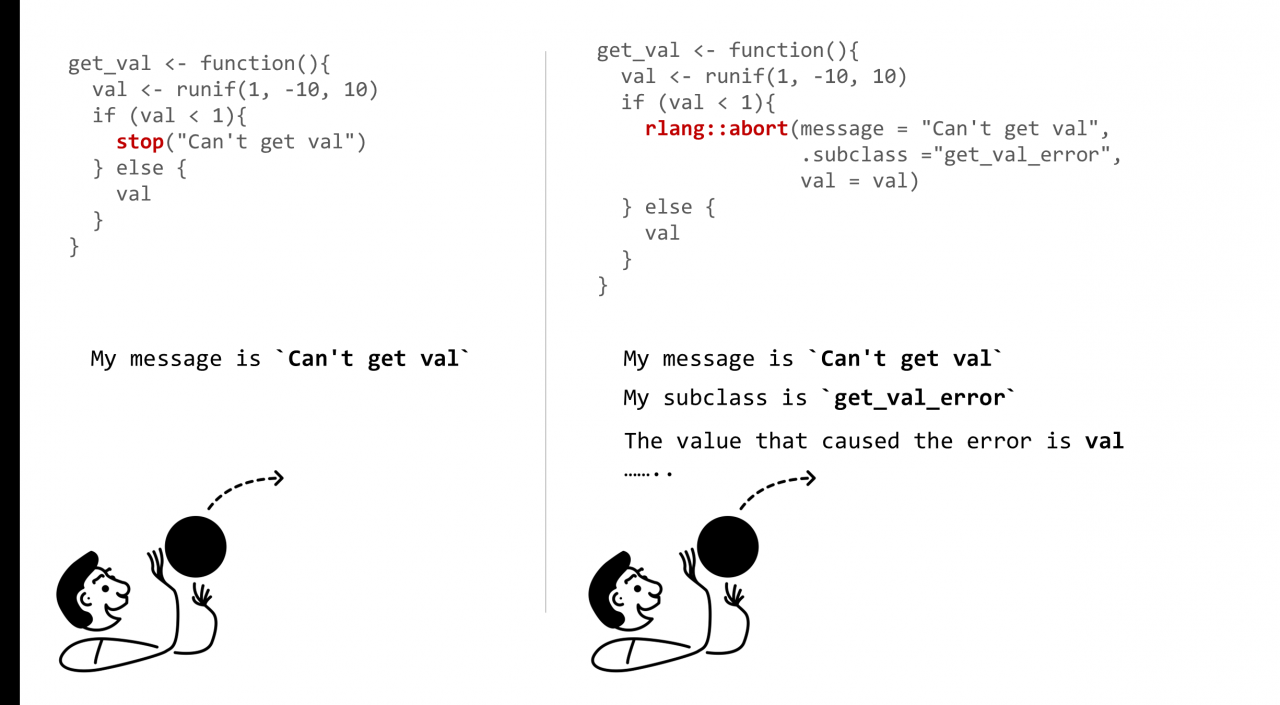# Error in *** : subscript out of bounds [How to Solve]

Error in *** : subscript out of bounds

Full error:Question:

The data is out of bounds. Except for others, who knows where it is? Do you ask memory? Who do you ask?

``````#make this example reproducible
set.seed(0)

#create matrix with 10 rows and 3 columns
x = matrix(data = sample.int(100, 30), nrow = 10, ncol = 3)

#print matrix
print(x)

#attempt to display 11th row of matrix
x[11, ]

#attempt to display 4th column of matrix
x[, 4]

#attempt to display value in 11th row and 4th column
x[11, 4]``````

Solution:

#

``````#display number of rows and columns in matrix
dim(x)``````

#

``````#display 10th row of matrix
x[10, ]

#display number of columns in matrix
ncol(x)

#display 3rd column of matrix
x[, 3]

#display value in 10th row and 3rd column of matrix
x[10, 3]``````

Full error Messages:
>
> #attempt to display 11th row of matrix
> x[11, ]
Error in x[11, ] : subscript out of bounds
>
> #attempt to display 4th column of matrix
> x[, 4]
Error in x[, 4] : subscript out of bounds
>
> #attempt to display value in 11th row and 4th column
> x[11, 4]
Error in x[11, 4] : subscript out of bounds
>